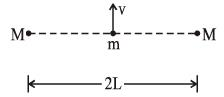Courses

# Test: Single Correct MCQs: Gravitation | JEE Advanced

## 16 Questions MCQ Test Physics For JEE | Test: Single Correct MCQs: Gravitation | JEE Advanced

Description
This mock test of Test: Single Correct MCQs: Gravitation | JEE Advanced for JEE helps you for every JEE entrance exam. This contains 16 Multiple Choice Questions for JEE Test: Single Correct MCQs: Gravitation | JEE Advanced (mcq) to study with solutions a complete question bank. The solved questions answers in this Test: Single Correct MCQs: Gravitation | JEE Advanced quiz give you a good mix of easy questions and tough questions. JEE students definitely take this Test: Single Correct MCQs: Gravitation | JEE Advanced exercise for a better result in the exam. You can find other Test: Single Correct MCQs: Gravitation | JEE Advanced extra questions, long questions & short questions for JEE on EduRev as well by searching above.
QUESTION: 1

### If the radius of the earth were to shrink by one percent, its mass remaining the same, the acceleration due to gravity on the earth’s surface would

Solution: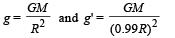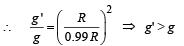QUESTION: 2

### If g is the acceleration due to gravity on the earth’s surface, the gain in the potential energy of an object of mass m raised from the surface of the  earth to a height equal to the radius R of the earth, is

Solution: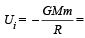Initial potential energy of the system.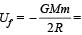Final P.E. of the system.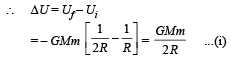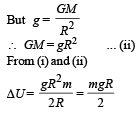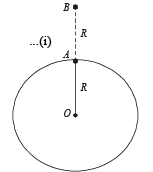QUESTION: 3

### If the distance between the earth and the sun were half its present value, the number of days in a year would have been

Solution:

According to Kepler's law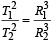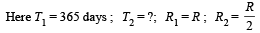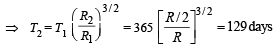QUESTION: 4

A geo-stationary satellite orbits around the earth in a circular orbit of radius 36,000km. Then, the time period of a spy satellite orbiting a few hundred km above the earth's surface (Rearth = 6,400km) will approximately be

Solution:

Note :  A satellite revolving near the earth's surface has a time period of 84.6 min.
We know that as the height increases, the time period increases. Thus the time period of the spy satellite should be slightly greater than 84.6 minutes.

∴ Ts = 2 hr

QUESTION: 5

A simple pendulum is oscillating without damping. When the displacement of the bob is less than maximum, its acceleration vector ar is correctly shown in :

Solution:

The components of acceleration are as shown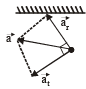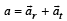The resultant of transverse and radial component of the acceleration is represented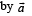QUESTION: 6

A binary star system consists of two stars A and B which have time period TA and TB, radius RA and RB and mass MA and MB. Then

Solution:

Note : The gravitational force of attraction between the stars will provide the necessary centripetal forces.
In this case angular velocity of both stars is the same.
Therefore time period remains the same.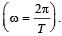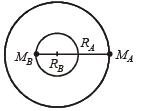QUESTION: 7

A spherically symmetric gravitational system of particles has a mass density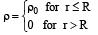where ρ0 is a constant. A test mass can undergo circular motion under the influence of the gravitational field of particles. Its speed v as a function of distance r (0 < r < ∞) from the centre of the system is represented by –

Solution:

For r > R

Force on the test mass m is F = m × | Eg |

Where Eg is the gravitational field intensity at the point of observation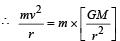where M is the total mass of the
spherical system.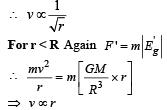QUESTION: 8

A thin uniform annular disc (see figure) of mass M has outer radius 4R and inner radius 3R. The work required to take a unit mass from point P on its axis to infinity is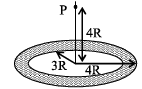Solution:

Let us consider a circular elemental area of radius x and thickness dx. The area of the shaded portion = 2πxdx.
Let dm be the mass of the shaded portion.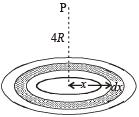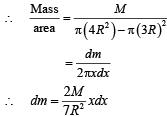The gravitational potential of the mass dm at P is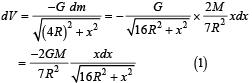Suppose 16R2 + x2 = t2
⇒  2xdx = 2tdt ⇒ xdx = tdt
Also for x = 3R, t = 5R
and for x = 4R,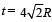On integrating equation (1),  taking the above limits, we get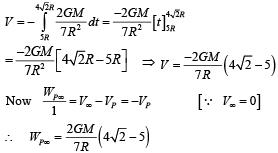QUESTION: 9

A satellite is moving with a constant speed ‘V’ in a circular orbit about the earth. An object of mass ‘m’ is ejected from the satellite such that it just escapes from the gravitational pull of the earth. At the time of its ejection, the kinetic energy of the object is

Solution:

V is the orbital velocity. If VC is the escape velocity then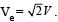The kinetic energy at the time of ejection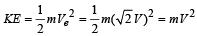QUESTION: 10

A planet of radiushas the same mass density as Earth. Scientists dig a well of depth R/5 onit and lower a wire of the same length and a linear mass density 10–3 kg m–1 into it. If the wire is not touching anywhere, the force applied at the top of the wire by a person holding it in place is (take the radius of Earth = 6 × 106 m and the acceleration due to gravity on Earth is 10 ms–2)

Solution: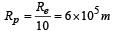The mass of the wire = 10–3 × 1.2 × 105 = 120 kg
Let gpM be the acceleration due to gravity at point M which is the mid point of the wire and is at a depth of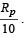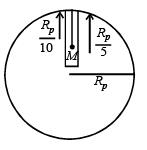Let gp be the acceleration due to gravity at the surface of the planet.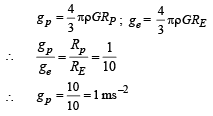and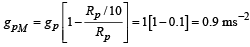∴ Force = mass of wire × gpM = 120 × 0.9 = 108 N

*Multiple options can be correct
QUESTION: 11

Imagine a light planet revolving around a very massive star in a circular orbit of radius R with a period of revolution T. If the gravitational force of attraction between the planet and the star is proportional to R–5/2

Solution:

KEY CONCEPT :  The centripetal force is provided by the gravitational force of attraction mRω2 = GMωR–5/2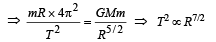*Multiple options can be correct
QUESTION: 12

A solid sphere of uniform density and radius 4 units is located with its centre at the origin O of coordinates. Two spheres of equal radii 1 unit, with their centres at A (–2, 0 ,0) and B (2, 0, 0) respectively, are taken out of the solid leaving behind spherical cavities as shown in fig Then :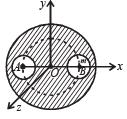Solution:

The gravitational field intensity at the point O is zero (as the cavities are symmetrical with respect to O). Now the force acting on a test mass m0 placed at O is given by

F = m0 E = m0 × 0 = 0

Now, y2 + z2 = 36 represents the equation of a circle with centre (0, 0, 0) and radius 6 units the plane of the circle is perpendicular to x-axis.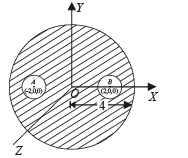Note :  Since the spherical mass distribution behaves as if the whole mass is at its centre (for a point outside on the sphere) and since all the points on the circle is equidistant from the centre of the sphere, the circle is a gravitational equipotential.
The same logic holds good for option (d).

*Multiple options can be correct
QUESTION: 13

The magnitudes of the gravitational field at distance r1 and r2 from the centre of a uniform sphere of radius R and mass m are F1 and F2 respectively. Then:

Solution:

For r > R, the gravitational field is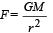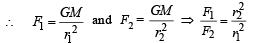For r < R, the gravitational field is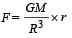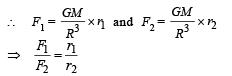*Multiple options can be correct
QUESTION: 14

A satellite S is moving in an elliptical orbit around the earth. The mass of the satellite is very small compared to the mass of the earth.

Solution:

Force on satellite is always towards earth, therefore, acceleration of satellite S is always directed towards centre of the earth. Net torque of this gravitational force F about centre of earth is zero. Therefore, angular momentum (both in magnitude and direction) of S about centre of earth is constant throughout. Since the force F is conservative in nature, therefore mechanical energy of satellite remains constant. Speed of S is maximum when it is nearest to earth and minimum when it is farthest.

*Multiple options can be correct
QUESTION: 15

Two spherical planets P and Q have the same uniform density ρ, masses MP and MQ and surface areas A and 4A respectively. A spherical planet R also has uniform density ρ and its mass is (MP + MQ). The escape velocities from the planets P, Q and R are VP, VQ and VR, respectively. Then

Solution:

Let the mass of P be m.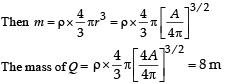∴ The mass of R = 9 m
If the radius of P = r
Then the radius of Q = 2r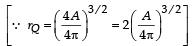and radius of R = 91/3r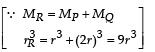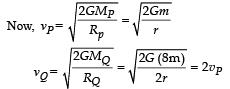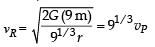*Multiple options can be correct
QUESTION: 16

Two bodies, each of mass M, are kept fixed with a separation 2L. A particle of mass m is projected from the midpoint of the line joining their centres, perpendicular to the line. The gravitational constant is G. The correct statement(s) is (are)

Solution: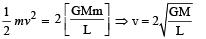The potential energy is a combined property of the three mass system. The kinetic energy of mass m is only its energy which decreases as it moves. (b) is the correct option.